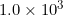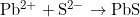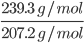×
Get Full Access to Introductory Chemistry - 5 Edition - Chapter 6 - Problem 70p
Get Full Access to Introductory Chemistry - 5 Edition - Chapter 6 - Problem 70p

×

# Lead is found in Earth's crust as several lead compounds. Calculate the mass (in kg) ofISBN: 9780321910295 34

## Solution for problem 70P Chapter 6

Introductory Chemistry | 5th Edition

• Textbook Solutions
• 2901 Step-by-step solutions solved by professors and subject experts
• Get 24/7 help from StudySoup virtual teaching assistantsIntroductory Chemistry | 5th Edition

4 5 1 404 Reviews
29
2
Problem 70P

Lead is found in Earth’s crust as several lead compounds. Calculate the mass (in kg) of each compound that contains $$1.0 \times 10^{3}$$ kg of lead.

(a) PbS (galena)

(b) $$\mathrm{PbCO}_{3}$$ (cerussite)

(c) $$\mathrm{PbSO}_{4}$$ (anglesite)

Equation Transcription:Text Transcription:

1.0 times 10^3

PbCO_3

PbSO_4

Step-by-Step Solution:

Step 1 of 3

Here, we have to calculate the mass of each compound that containskg of lead.

Molar mass of Pb = 207.2  g/mol

(a) PbSMolar ratio = 1:1

Molar mass of PbS = 239.3 g/mol

Mass of PbS = 1000 g= 1154.9 g = 1.1549 Kg

Thus, mass of PBs is  = 1.1549 Kg

Step 2 of 3

Step 3 of 3

## Discover and learn what students are asking

Calculus: Early Transcendental Functions : Basic Differentiation Rules and Rates of Change
?How Do You See It? Use the graph of f to answer each question. To print an enlarged copy of the graph, go to MathGraphs.com. (a) Betwee

Calculus: Early Transcendental Functions : Space Coordinates and Vectors in Space
?In Exercises 1-4, plot the points in the same three-dimensional coordinate system. (a) (2, 1, 3) (b) (-1, 2, 1)

Statistics: Informed Decisions Using Data : The Normal Approximation to the Binomial Probability Distribution
?In Problems 5–14, a discrete random variable is given. Assume the probability of the random variable will be approximated using the normal distributio

Unlock Textbook Solution